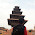### How to write PROLOG program for half adder?

In this problem, we are going to implement some logic (of course it is all about logic) that will run calculate the half adder sum and carry. We know has two inputs, and it will give sum and carry which looks like this:

X            Y                 Sum                 Carry
0            0                  0                      0
0            1                  1                      0
1            0                  1                      0
1            1                  0                      1

So, to implement sum we need XOR gate and to implement Carry we need AND gate.

Here is how we do it:

xORGate(0,0,0).
xORGate(0,0,0).
xORGate(0,0,0).
xORGate(0,0,0).

aNDGate(0,0,0).
aNDGate(0,0,0).
aNDGate(0,0,0).
aNDGate(0,0,0).

Now, you can run this code by giving the values of X and Y.
like this:
halfadder(1,1,S,C)  and the output will be
S=0
C=1

1.This comment has been removed by the author.

1.The half adder adds two single binary digits A and B. It has two outputs, sum (S) and carry (C). The Boolean logic for the sum (in this case S) will be A′B + AB′ i.e X-OR whereas for the carry (C) will be AB i.e AND.